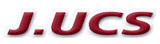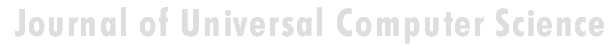Search
 Submission Procedure share: |available in:   PDF (128 kB) PS (38 kB)

get:
 Similar Docs BibTeX Write a comment
get:

DOI:   10.3217/jucs-004-02-0110

### Spline-Fourier Approximations of Discontinuous Waves

Roumen Anguelov (Vista University)

Abstract: In the Fourier series approximation of real functions discontinuities of the functions or their derivatives cause problems like Gibbs phenomenon or slow uniform convergence. In the case of a finite number of isolated discontinuities the problems can be to a large extend rectified by using periodic splines in the series. This modified Fourier series (Spline-Fourier series) is applied to the numerical solution of the wave equation (in periodic form) where discontinuities in the data functions or their derivatives appear quite often. The solution is sought in the form of a Spline-Fourier series about the space variable and close bounds are obtained using a certain iterative procedure of Newton type.

Keywords: Fourier hyper functoid, Validated numerics, Wave equation